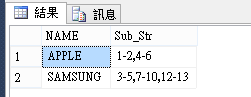#0

## SQL如何將連號合併為 ?-? 格式

NUM
－－－－－－－－－－
1
2
4
5
6

NAME　　　NUM
－－－－－－－－－－－
APPLE　　　1-2,4-6
SAMSUNG　3-5,7-10,12-13

### 2 個回答

0

``````declare @Tmp_Table1 as table(
NAME nvarchar(50)
,NUM int
)
insert into @Tmp_Table1
values('APPLE',1),('APPLE',2),('APPLE',4),('APPLE',6)
,('SAMSUNG',3),('SAMSUNG',5),('SAMSUNG',7),('SAMSUNG',10),('SAMSUNG',12),('SAMSUNG',13)

declare @Tmp_Table2 as table(
NAME nvarchar(50)
,Sub_Str nvarchar(Max)
)

declare @i int,@Max int,@NAME nvarchar(50),@Num_Str nvarchar(max)
declare @Sub_i int,@Sub_Max int
set @i = 1
set @Sub_i = 0
set @Num_Str = ''

select @Max = count(*)
from (
select NAME
from @Tmp_Table1 as a
group by NAME
) as k

while(@i <= @Max)
begin

select @Sub_Max = count(*)
from (
select Row_Number() Over(order by NAME) as Sort
,NAME
from (
select NAME
from @Tmp_Table1 as a
group by NAME
) as k
) as k
left join @Tmp_Table1 as b on k.NAME = b.NAME
where Sort = @i

select @NAME = k.NAME
,@Num_Str = @Num_Str + Convert(varchar,b.NUM)
+ (
case when @Sub_Max = Row_Number() Over(order by k.NAME)
then ''
else
case when Row_Number() Over(order by k.NAME) % 2 = 0 then ',' else '-' end
end
)
from (
select Row_Number() Over(order by NAME) as Sort
,NAME
from (
select NAME
from @Tmp_Table1 as a
group by NAME
) as k
) as k
left join @Tmp_Table1 as b on k.NAME = b.NAME
where Sort = @i

insert into @Tmp_Table2
values(@NAME,@Num_Str)

set @Num_Str = ''

set @i = @i + 1
end

select * from @Tmp_Table2

``````ken040714 iT邦新手 5 級 ‧ 2018-05-11 16:02:52 檢舉

1

#### 第一版 (SQL Fiddle連結):

• 沒有連續值情況要特別處理，舉例1、2、10
10沒有連續，所以我把它當成另外一個表格額為處理，最後標示為1-2、10
``````with CTE as (
select T1.num,T2.num n2,T3.num n3 from Table1 T1

--以下兩個join目的是取得每組最小跟最大的連貫值
left join Table1 T2 on T2.num = T1.num + 1
left join Table1 T3 on T3.num = T1.num - 1

--假如沒有null，代表是中間連貫值不需要考慮
--假如T2.num跟T3.num都是null代表沒有連續值
where T2.num is  null or T3.num is  null
)
--有連續值，按數字大小排序
,CTE2 as (
select num
,ROW_NUMBER() OVER(ORDER BY num) rank
from CTE
where not (n2 is null and n3 is null)
)
--連續組合-跟不連續union
,CTE3 as (
--有連續值組合
select convert(nvarchar(50),T1.num) + '-' + convert(nvarchar(50),T2.num) num
--藉由mod2來區分組最小值，跟最大值
from (select * from CTE2 where rank % 2 = 1) T1
left join (select * from CTE2 where rank % 2 = 0) T2 on T1.rank + 1 = T2.rank
union all
--沒有連續值
select convert(nvarchar(50),num) from CTE
where (n2 is null and n3 is null)
)
select STRING_AGG (num, ',') num from CTE3

``````ken040714 iT邦新手 5 級 ‧ 2018-05-11 15:02:36 檢舉

DB是SQL Server沒錯,強!!

ken040714 iT邦新手 5 級 ‧ 2018-05-11 16:02:25 檢舉